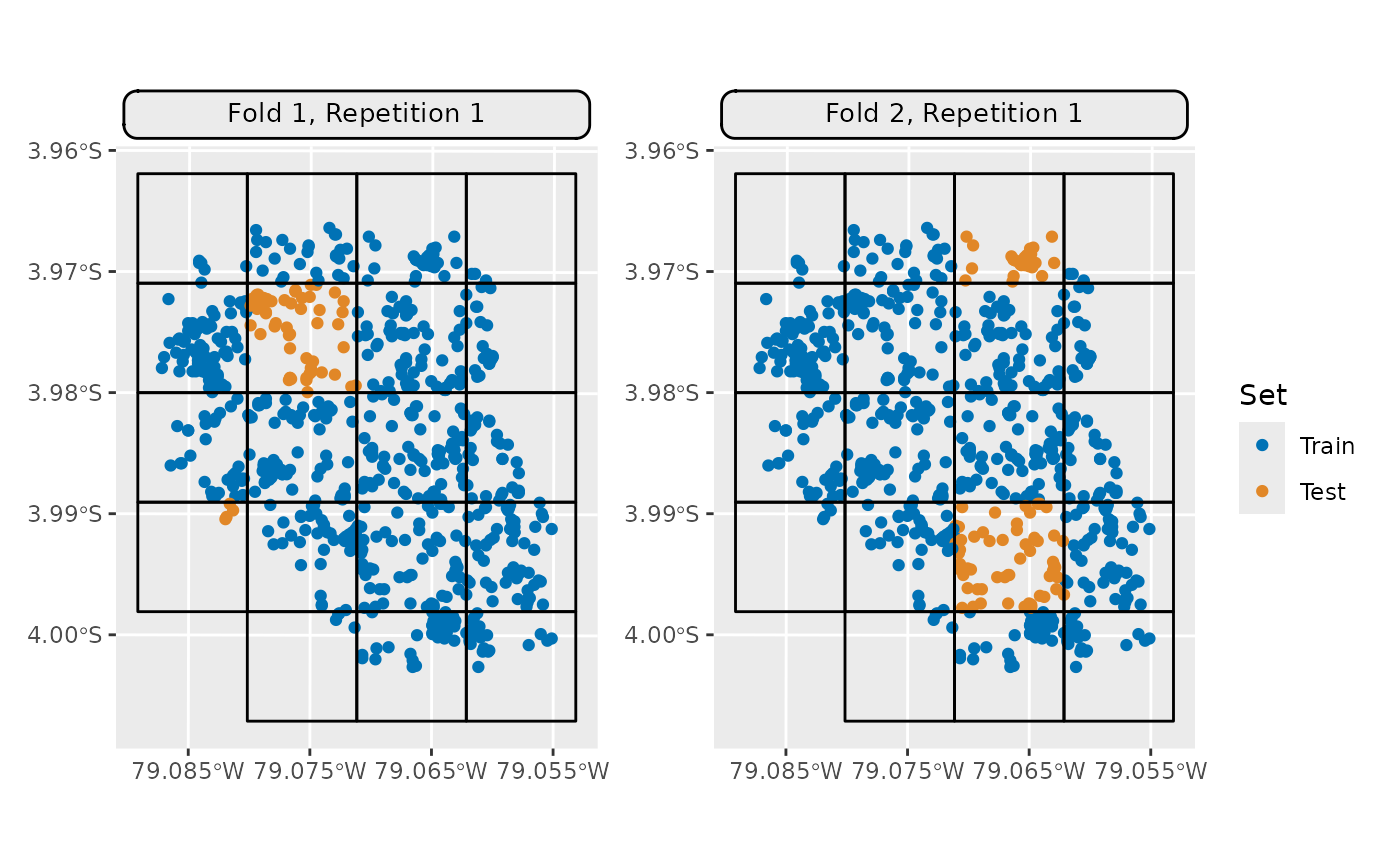Generic S3 plot() and autoplot() (ggplot2) methods to visualize mlr3 spatiotemporal resampling objects.

## Usage

# S3 method for ResamplingSpCVBlock
autoplot(
object,
fold_id = NULL,
plot_as_grid = TRUE,
train_color = "#0072B5",
test_color = "#E18727",
show_blocks = FALSE,
show_labels = FALSE,
sample_fold_n = NULL,
label_size = 2,
...
)

# S3 method for ResamplingRepeatedSpCVBlock
autoplot(
object,
fold_id = NULL,
repeats_id = 1,
plot_as_grid = TRUE,
train_color = "#0072B5",
test_color = "#E18727",
show_blocks = FALSE,
show_labels = FALSE,
sample_fold_n = NULL,
label_size = 2,
...
)

# S3 method for ResamplingSpCVBlock
plot(x, ...)

# S3 method for ResamplingRepeatedSpCVBlock
plot(x, ...)

## Arguments

object

[Resampling]
mlr3 spatial resampling object of class ResamplingSpCVBlock or ResamplingRepeatedSpCVBlock.

[TaskClassifST]/[TaskRegrST]

fold_id

[numeric]
Fold IDs to plot.

plot_as_grid

[logical(1)]
Should a gridded plot using via patchwork be created? If FALSE a list with of ggplot2 objects is returned. Only applies if a numeric vector is passed to argument fold_id.

train_color

[character(1)]
The color to use for the training set observations.

test_color

[character(1)]
The color to use for the test set observations.

show_blocks

[logical(1)]
Whether to show an overlay of the spatial blocks polygons.

show_labels

[logical(1)]
Whether to show an overlay of the spatial block IDs.

sample_fold_n

[integer]
Number of points in a random sample stratified over partitions. This argument aims to keep file sizes of resulting plots reasonable and reduce overplotting in dense datasets.

label_size

[numeric(1)]
Label size of block labels. Only applies for show_labels = TRUE.

...

Passed to geom_sf(). Helpful for adjusting point sizes and shapes.

repeats_id

[numeric]
Repetition ID to plot.

x

[Resampling]
mlr3 spatial resampling object. One of class ResamplingSpCVBuffer, ResamplingSpCVBlock, ResamplingSpCVCoords, ResamplingSpCVEnv.

## Value

ggplot() or list of ggplot2 objects.

## Details

By default a plot is returned; if fold_id is set, a gridded plot is created. If plot_as_grid = FALSE, a list of plot objects is returned. This can be used to align the plots individually.

When no single fold is selected, the ggsci::scale_color_ucscgb() palette is used to display all partitions. If you want to change the colors, call <plot> + <color-palette>().

• mlr3book chapter on "Spatiotemporal Visualization"

• autoplot.ResamplingSpCVBuffer()

• autoplot.ResamplingSpCVCoords()

• autoplot.ResamplingSpCVEnv()

• autoplot.ResamplingSpCVDisc()

• autoplot.ResamplingSpCVTiles()

• autoplot.ResamplingCV()

• autoplot.ResamplingSptCVCstf()

## Examples

# \donttest{
if (mlr3misc::require_namespaces(c("sf", "blockCV"), quietly = TRUE)) {
library(mlr3)
library(mlr3spatiotempcv)
resampling = rsmp("spcv_block", range = 1000L)

## list of ggplot2 resamplings
crs = 4326,
fold_id = c(1, 2), plot_as_grid = FALSE)

## Visualize all partitions
ggplot2::scale_x_continuous(breaks = seq(-79.085, -79.055, 0.01))

## Visualize the train/test split of a single fold
autoplot(resampling, task, fold_id = 1) +
ggplot2::scale_x_continuous(breaks = seq(-79.085, -79.055, 0.01))

## Visualize train/test splits of multiple folds
#> Warning: Ignoring unknown parameters: crs
#> Warning: Ignoring unknown parameters: crs